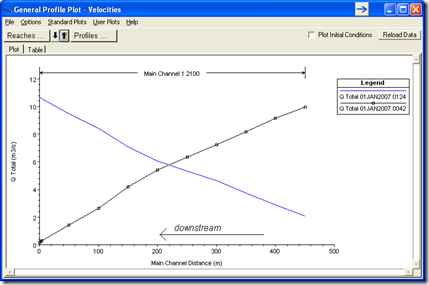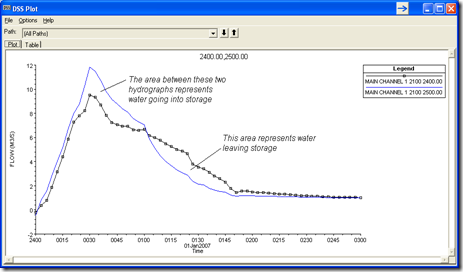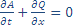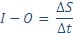## Thursday, June 28, 2012

### Q & A: Flow Attenuation

Written by Chris Goodell, P.E., D. WRE | WEST Consultants
Copyright © RASModel.com. 2012. All rights reserved.

Question
When running an unsteady flow model with a single inflow hydrograph, why does my discharge decrease in the downstream direction for a given output profile?

Answer
This is called flow attenuation.  You see this to varying degrees in all unsteady flow models and it is a real phenomenon.  The shallower the reach, or the wider the floodplain, the more pronounced this effect will be.  In very steep streams, you may not notice flow attenuation at all.
The physical process is as follows:  As the flood level rises, water moving downstream fills in available volume.  This volume is called storage.  Water going into storage is taken away from the flow going downstream and that is why you see a decrease in discharge as you move in the downstream direction.  Wider floodplains and shallower reaches have more available storage volume, which is why flow attenuation is pronounced under these situations.  Once the flood wave passes, and you are on the receding limb of the flood hydrograph, the water that had gone into storage now returns to the active discharge.  In this case you’ll see an increase in flow as you move in the downstream direction.  Notice in the figure below that the discharge at time 0042 (before the peak of the flood wave) decreases in the downstream direction, while the flow at time 0124 (after the peak of the flood wave) increases in the downstream direction.You can also see this effect when viewing hydrographs in the figure below from two cross sections, one upstream (River Station 2500) and one downstream (River Station 2400).  The attenuation of flow is the difference in peak discharge between these two hydrographs-in this case, about 2.3 cms.  The area between these two curves represents a volume of water.  The area to the left, where the upstream discharge is greater than that downstream discharge, represents water going into storage.  The area to the right, where the upstream discharge is less than the downstream discharge, represents water leaving storage.Attenuation is included in the conservation of mass equation, which is one of the two equations (the St. Venant equations) used to define the movement of water through a reach in HEC-RAS-the other being conservation of momentum.    From the HEC-RAS Hydraulic Reference Manual (Page 2-22), “Conservation of mass for a control volume states that the net rate of flow into the volume be equal to the rate of change of storage inside the volume.”    In other words, Inflow minus outflow equals the change in storage over time.  The equation is:where A = flow area, Q equals discharge, t = time, and x = length.
The discretized form of this is more practical to use and may be more familiar:Where I = Inflow to a discrete control volume, O = Outflow, DS = Change in Storage, Dt = time duration (i.e. time step).